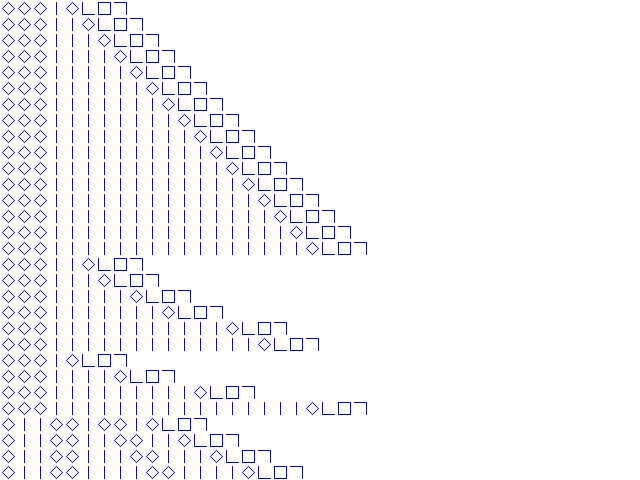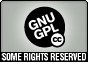## CosmicOS version 0.9

Latest version always available at CosmicOS homepage

 Message in compiled form
 The message consists of a long sequence of four basic symbols: ```00010223300011022330001110223300011110223300011111022330001111110223300011111110 22330001111111102233000111111111022330001111111111022330001111111111102233000111 11111111102233000111111111111102233000111111111111110223300011111111111111102233 00011111111111111110223300011022330001110223300011111022330001111111022330001111 11111110223300011111111111110223300010223300011110223300011111111102233000111111 11111111110223301100100102233011001100110223301100111001110223301100111100111102 23301100111110011111022330110011111100111111022330110011111110011111110223301100 ``` ...

 Message in graphical form
 This is a prettier way to view the message. This is not the form in which the message would be transmitted, just a more convenient form for a casual human reader to skim.... ... (click for full message)

 Message in human-readable form
This form of the message is close to the actual "source code" of the message, and should be much easier to read (especially if you are a programmer).

Click here for complete message - warning, this is a very large document, you are probably better off looking at individual sections:

 Sect. Comment Type 0 introduce numbers (in unary notation) (MATH) 1 introduce equality for unary numbers (MATH) 2 now introduce other relational operators (MATH) 3 introduce the NOT logical operator (MATH) 4 introduce addition (MATH) 5 introduce subtraction (MATH) 6 introduce multiplication (MATH) 7 introduce a simple form of binary notation (MATH) 8 show local assignment (MATH) 9 demonstrate existence of memory (MATH) 10 use equality for truth values (MATH) 11 show mechanisms for branching (MATH) 12 introduce the AND logical operator (MATH) 13 introduce the OR logical operator (MATH) 14 illustrate pairs (MATH) 15 introduce mutable objects, and side-effects (MATH) 16 illustrate lists and some list operators (MATH) 17 describe changes to the implicit interpreter to allow new special forms (HACK) 18 introduce sugar for let (MATH) 19 build up functions of several variables (MATH) 20 show map function for applying a function across the elements of a list (MATH) 21 end of part 1, start of part 2 (NOTE) 22 show an example of recursive evaluation (MATH) 23 some pure lambda calculus definitions - optional (MATH) 24 introduce universal quantifier (MATH) 25 introduce existential quantifier (MATH) 26 introduce logical implication (MATH) 27 introduce sets and set membership (MATH) 28 introduce graph structures (MATH) 29 show how to execute a sequence of instructions (MATH) 30 introduce environment / hashmap structure (MATH) 31 introduce simple mutable structures (OBJECT) 32 introduce method handler wrappers (OBJECT) 33 introduce turing machine model (TURING) 34 introduce simple form of typing, for ease of documentation. (OBJECT) 35 an example object -- a 2D point (OBJECT) 36 an example object -- a container (OBJECT) 37 expressing inheritance (OBJECT) 38 adding a special form for classes (OBJECT) 39 wrapper class for cells (OBJECT) 40 playing around with doors and rooms (MUD) 41 end of part 2, start of part 3 (NOTE) 42 simulating unless gates (GATE) 43 testing alternate primer based on gates: COS_NOT circuit (GATE) 44 testing alternate primer based on gates: COS_AND circuit (GATE) 45 testing alternate primer based on gates: COS_OR circuit (GATE) 46 testing alternate primer based on gates: COS_NOR circuit (GATE) 47 testing alternate primer based on gates: COS_OSC circuit (GATE) 48 testing alternate primer based on gates: COS_SR circuit (GATE) 49 testing alternate primer based on gates: COS_D circuit (GATE) 50 probing networks of unless gates (GATE) 51 end of part 3, start of part 4 (NOTE) 52 a mechanism for referring to parts of the message (SELF) 53 some preparatory work for integrating with Java code (JAVA) 54 class translation 'COS_JavaTest' (JAVA) 55 check that automatic conversion is workable (JAVA) 56 another simple little text-adventure space (MUD) 57 native implementation of a Java list, hash classes (JAVA) 58 testing the JList class (JAVA) 59 basic iterator implementation (JAVA) 60 class translation 'COS_JDoor' (JAVA) 61 class translation 'COS_JThing' (JAVA) 62 class translation 'COS_JRoom' (JAVA) 63 class translation 'COS_JNamed' (JAVA) 64 class translation 'COS_JWorld' (JAVA) 65 class translation 'COS_JRobo' (JAVA) 66 test JRoom, JDoor, JThing, etc (JAVA)

 Message as a single imageThis is just for fun. The entire image is embedded in a single image, with each pixel corresponding to one symbol in the message. The image reads diagonally, starting at the top left. It is handy for spotting phase changes in the message (e.g. the transition from using unary to binary, or - more importantly - bugs). Zeros are greenish, ones are reddish, parentheses (symbols 2 and 3) are different shades of blue.

 Version notes
 Estimate of message entropy in compiled form: 25.3 kB. There are bugs and rough edges in the message. Please be forgiving. It'll all get fixed in an instant of galactic time... Structure of message:The message consists of a sequence of statements that can be evaluated, and each statement evaluates to true.The message can refer to and change its own syntax.A core set of operators are introduced by showing examples of their use.Once a sufficient set of operators is available, most new concepts are introduced both by examples and a definition in terms of what is already known.The message is more like a programming language than a human language, and is related to Brian McConnell's notion of ACETI. The goal is to transmit simulations whose behavior can be examined and discussed, and to introduce higher-level notions in terms of those simulations. The advantage of using a programming-code-like language is that the reader can play with hypothethicals at any time, and experiment with evaluating alternative statements that are not in the message.The generated message currently consists of a sequence of 4 symbols.``` number symbol meaning 0 . binary digit zero 1 : binary digit one 2 ( marks beginning of an expression 3 ) marks end of an expression ```And two pseudo-symbols coded using the above:``` sequence symbol meaning .() / opens an implicit paren, which will close at next paren (A B / C / D) is another way to write (A B (C (D))) This greatly simplifies complex expressions. (()) ; marks end of sentence ```Numbers are encoded as binary digits between parentheses, e.g. (:::.) is 1110 base 2 which is 14 in decimal. A set of numbers between parentheses constitutes an expression. Expressions can be nested. Expressions followed by a semicolon should evaluate to be true, once the rules for evaluation have been introduced.In the human-readable form of the message, decimal numbers can be used. There are converted to binary. Identifiers can also be used. Identifiers are mapped onto arbitrarily assigned numbers. In the message, there is nothing to distinguish identifiers from numbers. The actual language is carefully constructed so that this distinction is never necessary.Here are the operations currently introduced in the message by example alone, rather than by definition. equals, greater than, less than, not addition, multiplication, subtraction, (division) assign, define, "?", "number?" make-cell, set!, get! primer translate I'm gradually reducing this set. There are some obvious redundancies that could be removed quite easily right now, but with a cost to comprehension. reasonably readable form of message checker for the message (scheme code) and the current results of checking intermediate form of message final form of message. Archive cosmic-0-9.tgz of all files.The CosmicOS version 0.9 software is licensed under the CC-GNU GPL.The CosmicOS version 0.9 webpages are licensed under a Creative Commons Attribution-ShareAlike 2.5 License.